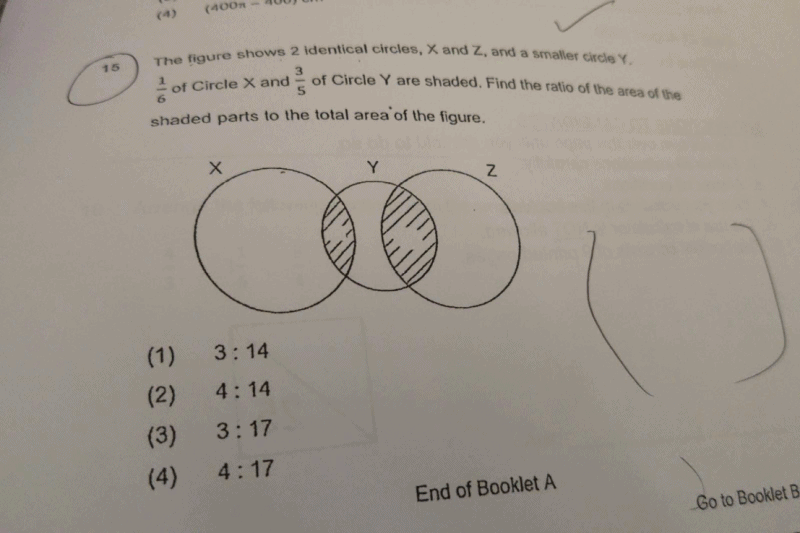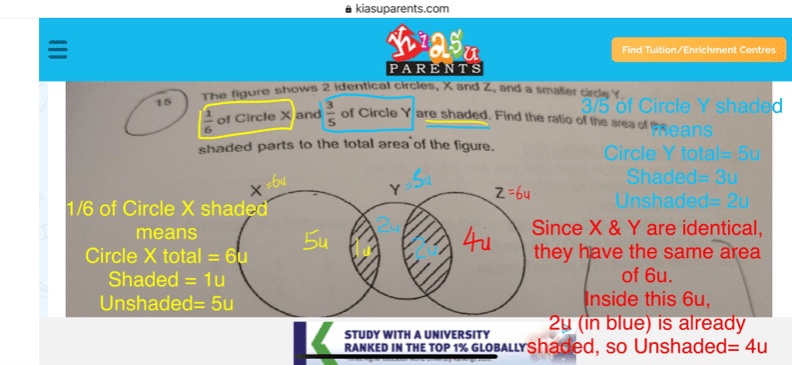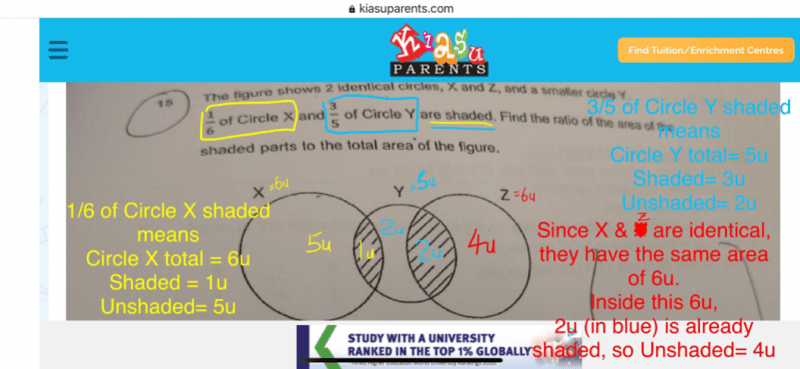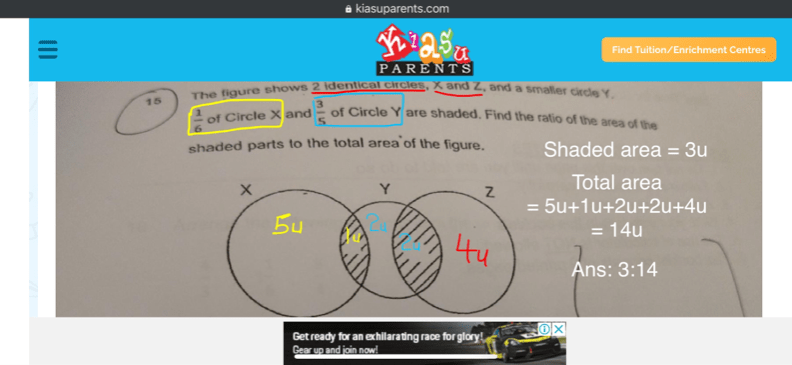# Question2 Replies 0 Likes ✔Accepted Answer

Hi Math Investigator,

The shaded area in Circle X has the same area in Circle Y. This shaded area has the same shape and size whether I put it in Circle X or Circle Y.  1u of shaded X is equal to 1u of shaded Y (Take note that the equal parts refer to the shaded area, not total area)

The difference is in the TOTAL AREA of both circles. Meaning to say, their individual TOTAL SIZE is different, but the part where they OVERLAP is the same, has the same size same shape, hence sharing the SAME AREA.

Hope this clarifies

Thank you for your reply. I am afraid that you misunderstood my question. 1 unit of X may be larger than 1 unit of Y. Hence, we can’t conclude that the shaded part of Z is 2 units.

Lets say X = 60 cm2 , Y = 45 cm2 , Z = 60 cm2

In this situation, the shaded part of X is 10 cm2 (1/6 x 60 =10) the total shaded of Y is 27 cm2 (3/5 x 45), then the remaining shaded part of Z is 17 cm2 (27 – 10). As a result, the ratio will not work.

I tried solving this question and got stuck because of this problem. In order for a particular math concept to apply, it must work in all situations.

Quote: “Lets say X = 60 cm2 , Y = 45 cm2 , Z = 60 cm2”

the ratio of X : Y is 6 : 5 , which is given and stated in the question “1/6 of X & 3/5 of Y”(not 6 : 4.5)

so it should be said “X=60cm2, Y=50cm2, Z=60cm2”

now try doing again with the correct ratio. 😉

Seems like I can’t seem to let you understand what my problem is. Let me try one last time.

When the question simply say 1/6 of Circle X and 3/5 of Circle Y are shaded, we cannot simply conclude that the ratio of Circle X to Circle Y is 6 : 5

The reason is because we do not have enough information to prove that 1 unit of Circle X is equal to 1 unit of Circle Y

Just like if I were to simply just say 1/6 of the boys and 3/5 of the girls wear spectacles, we cannot simply immediately conclude that the ratio of boys to girls must be 6 : 5  for the same reason.

I believe that there is an error in this question.

0 Replies 0 LikesHi Math Investigator & helpforpsle,

I made a typo error in the red words.

Area of X should be equal to Area of Z, not Area of Y.

The area of these 2 circles are the same because the first sentence in the question stated “…. 2 identical circles, X and Z,……”

Apologies for the mistake.

Here is the corrected image.

0 Replies 0 Likes

Thank you so much I got it now🙏

Welcome. 😊

0 Replies 0 LikesCan you pls explain, how the unshaded part of circle z is 4u as the fraction says 3/5 ? Also unshaded part of small circle is 2u?2 Replies 0 Likes ✔Accepted Answer

Hi Math Investigator,

The shaded area in Circle X has the same area in Circle Y. This shaded area has the same shape and size whether I put it in Circle X or Circle Y.  1u of shaded X is equal to 1u of shaded Y (Take note that the equal parts refer to the shaded area, not total area)

The difference is in the TOTAL AREA of both circles. Meaning to say, their individual TOTAL SIZE is different, but the part where they OVERLAP is the same, has the same size same shape, hence sharing the SAME AREA.

Hope this clarifies

Thank you for your reply. I am afraid that you misunderstood my question. 1 unit of X may be larger than 1 unit of Y. Hence, we can’t conclude that the shaded part of Z is 2 units.

Lets say X = 60 cm2 , Y = 45 cm2 , Z = 60 cm2

In this situation, the shaded part of X is 10 cm2 (1/6 x 60 =10) the total shaded of Y is 27 cm2 (3/5 x 45), then the remaining shaded part of Z is 17 cm2 (27 – 10). As a result, the ratio will not work.

I tried solving this question and got stuck because of this problem. In order for a particular math concept to apply, it must work in all situations.

Quote: “Lets say X = 60 cm2 , Y = 45 cm2 , Z = 60 cm2”

the ratio of X : Y is 6 : 5 , which is given and stated in the question “1/6 of X & 3/5 of Y”(not 6 : 4.5)

so it should be said “X=60cm2, Y=50cm2, Z=60cm2”

now try doing again with the correct ratio. 😉

Seems like I can’t seem to let you understand what my problem is. Let me try one last time.

When the question simply say 1/6 of Circle X and 3/5 of Circle Y are shaded, we cannot simply conclude that the ratio of Circle X to Circle Y is 6 : 5

The reason is because we do not have enough information to prove that 1 unit of Circle X is equal to 1 unit of Circle Y

Just like if I were to simply just say 1/6 of the boys and 3/5 of the girls wear spectacles, we cannot simply immediately conclude that the ratio of boys to girls must be 6 : 5  for the same reason.

I believe that there is an error in this question.

0 Replies 0 LikesHi Math Investigator & helpforpsle,

I made a typo error in the red words.

Area of X should be equal to Area of Z, not Area of Y.

The area of these 2 circles are the same because the first sentence in the question stated “…. 2 identical circles, X and Z,……”

Apologies for the mistake.

Here is the corrected image.

0 Replies 0 Likes

Thank you so much I got it now🙏